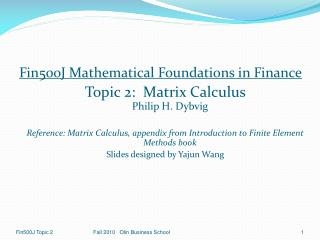DownloadDownload PresentationFin500J Mathematical Foundations in Finance Topic 2: Matrix Calculus Philip H. Dybvig

# Fin500J Mathematical Foundations in Finance Topic 2: Matrix Calculus Philip H. Dybvig

Télécharger la présentation## Fin500J Mathematical Foundations in Finance Topic 2: Matrix Calculus Philip H. Dybvig

- - - - - - - - - - - - - - - - - - - - - - - - - - - E N D - - - - - - - - - - - - - - - - - - - - - - - - - - -
##### Presentation Transcript

1. Fin500J Mathematical Foundations in Finance Topic 2: Matrix Calculus Philip H. Dybvig Reference: Matrix Calculus, appendix from Introduction to Finite Element Methods book Slides designed by Yajun Wang Fall 2010 Olin Business School

2. Outline • The Derivatives of Vector Functions • The Chain Rule for Vector Functions Fall 2010 Olin Business School

3. 1 The Derivatives of Vector Functions Fall 2010 Olin Business School

4. 1.1 Derivative of Vector with Respect to Vector Fall 2010 Olin Business School

5. 1.2 Derivative of a Scalar with Respect to Vector If y is a scalar It is also called the gradient of y with respect to a vector variable x, denoted by . 1.3 Derivative of Vector with Respect to Scalar Fall 2010 Olin Business School

6. Example 1 Given and Fall 2010 Olin Business School

7. In Matlab >> syms x1 x2 x3 real; >> y1=x1^2-x2; >> y2=x3^2+3*x2; >> J = jacobian([y1;y2], [x1 x2 x3]) J = [ 2*x1, -1, 0] [ 0, 3, 2*x3] Note: Matlab defines the derivatives as the transposes of those given in this lecture. >> J' ans = [ 2*x1, 0] [ -1, 3] [ 0, 2*x3] Fall 2010 Olin Business School

8. Some useful vector derivative formulas Homework Fall 2010 Olin Business School

9. Important Property of Quadratic Form xTCx Proof: If C is symmetric, Fall 2010 Olin Business School

10. 2 The Chain Rule for Vector Functions Let where z is a function of y, which is in turn a function of x, we can write Each entry of this matrix may be expanded as Fall 2010 Olin Business School

11. The Chain Rule for Vector Functions (Cont.) Then On transposing both sides, we finally obtain This is the chain rule for vectors (different from the conventional chain rule of calculus, the chain of matrices builds toward the left) Fall 2010 Olin Business School

12. Example 2 x, y are as in Example 1 and z is a function of y defined as Fall 2010 Olin Business School

13. In Matlab >> z1=y1^2-2*y2; >> z2=y2^2-y1; >> z3=y1^2+y2^2; >> z4=2*y1+y2; >> Jzx=jacobian([z1; z2; z3; z4],[x1 x2 x3]) Jzx = [ 4*(x1^2-x2)*x1, -2*x1^2+2*x2-6, -4*x3] [ -2*x1, 6*x3^2+18*x2+1, 4*(x3^2+3*x2)*x3] [ 4*(x1^2-x2)*x1, -2*x1^2+20*x2+6*x3^2, 4*(x3^2+3*x2)*x3] [ 4*x1, 1, 2*x3] >> Jzx’ ans = [ 4*(x1^2-x2)*x1, -2*x1, 4*(x1^2-x2)*x1, 4*x1] [ -2*x1^2+2*x2-6, 6*x3^2+18*x2+1, -2*x1^2+20*x2+6*x3^2, 1] [ -4*x3, 4*(x3^2+3*x2)*x3, 4*(x3^2+3*x2)*x3, 2*x3] Fall 2010 Olin Business School